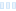Here's a fractal with 100% of points Inside; no points reach the bailout criterion. The Statistics tab says that the Min and Max iterations are both zero. I would have expected that both Min and Max would be set to #maxiter.

This is either a bug or a feature.

Here's an example:
/>test {
fractal:
title="test" width=800 height=600 layers=1
credits="Jim Blue;10/5/2022" antialiasing=yes
layer:
caption="Background" opacity=100 method=multipass
mapping:
center=3.005/1.935 magn=16
formula:
entry="Mandelbrot" p_start=0/0 p_power=-1.5/0 p_bailout=1e10
inside:
transfer=none solid=4285464576
outside:
density=.1 transfer=linear solid=4278233600 filename="Standard.ucl"
entry="Smooth" p_power=2/0 p_bailout=128.0
color=8716288 index=100 color=16121855 index=200 color=46591
index=300 color=156
opacity:
smooth=no index=0 opacity=255
}

Here&#039;s a fractal with 100% of points Inside; no points reach the bailout criterion. The Statistics tab says that the Min and Max iterations are both zero. I would have expected that both Min and Max would be set to #maxiter. This is either a bug or a feature. Here&#039;s an example: test { fractal: title=&quot;test&quot; width=800 height=600 layers=1 credits=&quot;Jim Blue;10/5/2022&quot; antialiasing=yes layer: caption=&quot;Background&quot; opacity=100 method=multipass mapping: center=3.005/1.935 magn=16 formula: maxiter=100 percheck=off adjust=yes filename=&quot;Standard.ufm&quot; entry=&quot;Mandelbrot&quot; p_start=0/0 p_power=-1.5/0 p_bailout=1e10 inside: transfer=none solid=4285464576 outside: density=.1 transfer=linear solid=4278233600 filename=&quot;Standard.ucl&quot; entry=&quot;Smooth&quot; p_power=2/0 p_bailout=128.0 gradient: comments=&quot;Default Ultra Fractal gradient.&quot; smooth=yes index=0 color=8716288 index=100 color=16121855 index=200 color=46591 index=300 color=156 opacity: smooth=no index=0 opacity=255 }

0
22
views
0
replies
1
followers
live preview
Enter at least 10 characters.
WARNING: You mentioned %MENTIONS%, but they cannot see this message and will not be notifiedSaving...
Saved
All posts under this topic will be deleted ?
Pending draft ... Click to resume editing